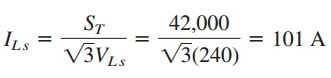# Easy Understanding Three Phase Transformer Equivalent CircuitThree phase transformer is mainly used for high power transmission.

To meet the demand for three phase power transmission, transformer connections compatible with three-phase operations are needed.

We can achieve the transformer connections in two ways: by connecting three single-phase transformers, thereby forming a so-called transformer bank, or by using a special three-phase transformer.

Contents

For the same kVA rating, a three-phase transformer is always smaller and cheaper than three single-phase transformers. When single-phase transformers are used, one must ensure that they have the same turns ratio n to achieve a balanced three-phase system.

## Three Phase Transformer

There are four standard ways of connecting three single-phase transformers or a three-phase transformer for three-phase operations: Y-Y, Δ-Δ, Y-Δ, and Δ-Y.

For any of the four connections, the total apparent power ST, real power PT, and reactive power QT are obtained as

where VL and IL are, respectively, equal to the line voltage VLp and the line current ILp for the primary side, or the line voltage VLs and the line current ILs for the secondary side.

Notice from Equation.(1) that for each of the four connections, VLsILs = VLpILp, since power must be conserved in an ideal transformer.

For the Y-Y connection in Figure.(1), the line voltage VLp at the primary side, the line voltage VLs on the secondary side, the line current ILp on the primary side, and the line current ILs on the secondary side is related to the transformer per phase turns ratio n as

For the Δ-Δ connection in Figure.(2), Equation.(2) also applies for the line voltages and line currents.

This connection is unique in the sense that if one of the transformers is removed for repair or maintenance, the other two form an open delta, which can provide three-phase voltages at a reduced level of the original three-phase transformer.

For the Y-Δ connection in Figure.(3), there is a factor of √3 arising from the line-phase values in addition to the transformer per phase turns ratio n. Thus,

Similarly, for the Δ-Y connection in Figure.(4),

Thus,

## Three Phase Transformer Equivalent Circuit Example

The 42-kVA balanced load depicted in Figure.(5) is supplied by a three phase transformer. (a) Determine the type of transformer connections. (b) Find the line voltage and current on the primary side. (c) Determine the kVA rating of each transformer used in the transformer bank. Assume that the transformers are ideal.

Solution:
(a) A careful observation of Figure.(5) shows that the primary side is Y-connected, while the secondary side is Δ-connected. Thus, the three phase transformer is Y-Δ, similar to the one shown in Figure.(2).

(b) Given a load with total apparent power ST = 42 kVA, the turns ratio n = 5, and the secondary line voltage VLs = 240 V, we can find the secondary line current using Equation.(1a), byFrom Equation.(3)(c) Because the load is balanced, each transformer equally shares the total load and since there are no losses (assuming ideal transformers), the kVA rating of each transformer is S = ST/3 = 14 kVA.

Alternatively, the transformer rating can be determined by the product of the phase current and phase voltage of the primary or secondary side.

For the primary side, for example, we have a delta connection, so that the phase voltage is the same as the line voltage of 240 V, while the phase current is ILp/√3 = 58.34 A. Hence, S = 240 × 58.34 = 14 kVA.